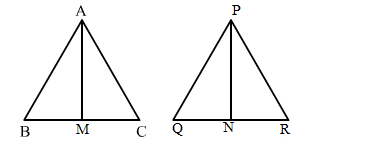# The areas of two similar triangles are 100 cm2 and 64 cm2 respectively.

Question:

The areas of two similar triangles are 100 cm2 and 64 cm2 respectively. If a median of the similar triangle is 5.6 cm, find the corresponding median of the other.

Solution:

Let the two triangles be ABC and PQR with medians AM and PN, respectively.Therefore, the ratio of areas of two similar triangles will be equal to the ratio of squares of their corresponding medians.

$\therefore \frac{\operatorname{ar}(\Delta A B C)}{\operatorname{ar}(\Delta P Q R)}=\frac{A M^{2}}{P N^{2}}$

$\Rightarrow \frac{64}{100}=\frac{5.6^{2}}{P N^{2}}$

$\Rightarrow P N^{2}=\frac{64}{100} \times 5.6^{2}$

$\Rightarrow P N^{2}=\sqrt{\frac{100}{64} \times 5.6 \times 5.6}$

$=7 \mathrm{~cm}$

Hence, the median of the larger triangle is 7 cm.